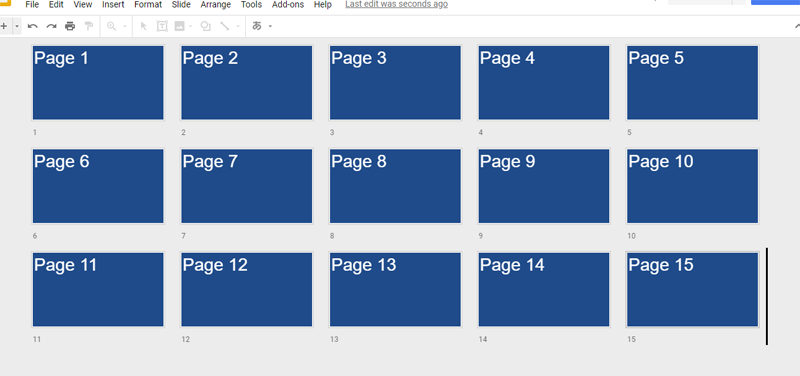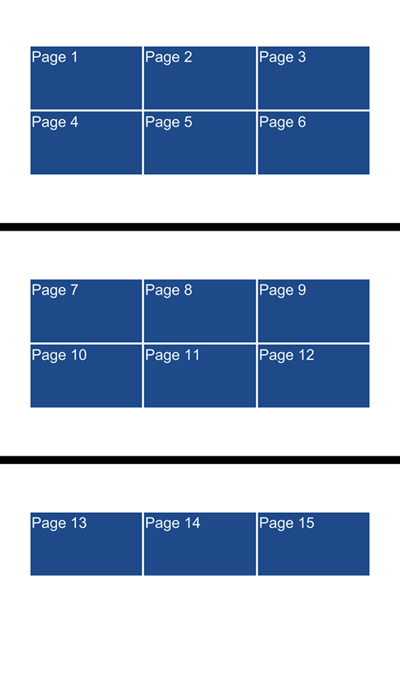# Summarizing Slides as Thumbnails

Gists

This is a sample script for summarizing Slides as thumbnails. For example, it supposes a Slides including 15 pages. When this script is run, it summarizes 6 pages to one page as images. I created this because there are no methods for directly achieving this. This is useful for myself. If this is also useful for you, I’m glad.

The flow of this workaround is as follows.

### Flow:

1. Copy the original Slides file as a temporary file.
2. Retrieve images of all slides.
• When the Slides file is exported to PNG file, the top page is exported as PNG file. I used this.
3. Put and arrange the retrieved images to the temporary file.
• When the image is inserted to a slide, retrieve the size and change the size, then put the image to the calculated position.

### Sample script 1:

function myFunction() {
var id = "### file ID ###"; // file ID of original Slides
var col = 3; // Number of columns
var row = 2; // Number of rows
var wsize = 200; // Size of width of each image (pixels)
var sep = 5; // Space of each image (pexels)

// Create temporary file
var originalFile = DriveApp.getFileById(id);
var tempFile = originalFile.makeCopy();
var idt = tempFile.getId();

// Retrieve slides as images
var s = SlidesApp.openById(idt);
var slides = s.getSlides();
var accessToken = ScriptApp.getOAuthToken();
var baseUrl = "https://docs.google.com/presentation/d/" + idt + "/export/";
var url = baseUrl + "png?access_token=" + accessToken;
var blobs = slides.map(function(e) {
var blob = UrlFetchApp.fetch(url).getBlob();
slides.remove();
s.saveAndClose();
s = SlidesApp.openById(idt);
slides = s.getSlides();
return blob;
});

// Put images
var ph = s.getPageHeight();
var pw = s.getPageWidth();
var leftOffset = (pw - ((wsize * col) + (sep * (col - 1)))) / 2;
if (leftOffset < 0) throw new Error("Images are sticking out from a slide.");
var len = col * row;
var loops = Math.ceil(blobs.length / (col * row));
for (var loop = 0; loop < loops; loop++) {
var ns = s.insertSlide(loop);
var topOffset, top;
var left = leftOffset;
for (var i = len * loop; i < len + (len * loop); i++) {
if (i === blobs.length) break;
var image = ns.insertImage(blobs[i]);
var w = image.getWidth();
var h = image.getHeight();
var hsize = h * wsize / w;
if (i === 0 || i % len === 0) {
topOffset = (ph - ((hsize * row) + sep)) / 2;
if (topOffset < 0) throw new Error("Images are sticking out from a slide.");
top = topOffset;
}
image.setWidth(wsize).setHeight(hsize).setTop(top).setLeft(left).getObjectId();
if (i === col - 1 + (loop * len)) {
top = topOffset + hsize + sep;
left = leftOffset;
} else {
left += wsize + sep;
}
}
}
s.saveAndClose();
}


### Sample script 2:

When you use this script, please enable Slides API at API console.

function myFunction() {
var id = "### file ID ###"; // file ID of original Slides
var col = 3; // Number of columns
var row = 2; // Number of rows
var wsize = 200; // Size of width of each image (pixels)
var sep = 5; // Space of each image (pexels)

// Retrieve slides as images
var originalFile = SlidesApp.openById(id);
var accessToken = ScriptApp.getOAuthToken();
var pageObjectIds = originalFile.getSlides().map(function(e) {return e.getObjectId()});
var reqUrls = pageObjectIds.map(function(pageObjectId) {
return {
method: "get",
url: "https://slides.googleapis.com/v1/presentations/" + id + "/pages/" + pageObjectId + "/thumbnail?access_token=" + accessToken,
};
});
var reqBlobs = UrlFetchApp.fetchAll(reqUrls).map(function(e) {
var r = JSON.parse(e);
return {
method: "get",
url: r.contentUrl,
};
});
var blobs = UrlFetchApp.fetchAll(reqBlobs).map(function(e) {return e.getBlob()});

// Create a temporary Slides and put images
var s = SlidesApp.create("temporarySlides");
s.getSlides().remove();
var idt = s.getId();
var ph = s.getPageHeight();
var pw = s.getPageWidth();
var leftOffset = (pw - ((wsize * col) + (sep * (col - 1)))) / 2;
if (leftOffset < 0) throw new Error("Images are sticking out from a slide.");
var len = col * row;
var loops = Math.ceil(blobs.length / (col * row));
for (var loop = 0; loop < loops; loop++) {
var ns = s.insertSlide(loop);
var topOffset, top;
var left = leftOffset;
for (var i = len * loop; i < len + (len * loop); i++) {
if (i === blobs.length) break;
var image = ns.insertImage(blobs[i]);
var w = image.getWidth();
var h = image.getHeight();
var hsize = h * wsize / w;
if (i === 0 || i % len === 0) {
topOffset = (ph - ((hsize * row) + sep)) / 2;
if (topOffset < 0) throw new Error("Images are sticking out from a slide.");
top = topOffset;
}
image.setWidth(wsize).setHeight(hsize).setTop(top).setLeft(left).getObjectId();
if (i === col - 1 + (loop * len)) {
top = topOffset + hsize + sep;
left = leftOffset;
} else {
left += wsize + sep;
}
}
}
s.saveAndClose();

// Export PDF file
var urlPdf = "https://docs.google.com/presentation/d/" + idt + "/export/" + "pdf?access_token=" + accessToken;
var pdf = UrlFetchApp.fetch(urlPdf).getBlob();
DriveApp.createFile(pdf.setName(originalFile.getName() + ".pdf"));
DriveApp.getFileById(idt).setTrashed(true);
}


## Input:

This is the original Slides.## Output:

This is the exported PDF file.## Note:

• This script was posted to https://stackoverflow.com/a/53775150/7108653.
• Both samples can obtain the same result. So please select one of them.
• The process cost of “Sample script 2” is lower than that of “Sample script 1”, because at “Sample script 2”, all thumbnail images are retrieved using the fetchAll method.
• I think that when “Sample script 1” is used, the thumbnail list can be also created for other Google Docs (Spreadsheet and Document).